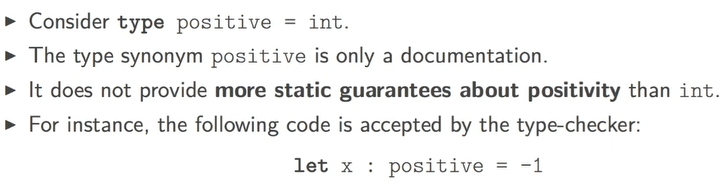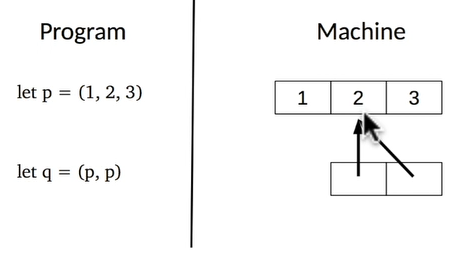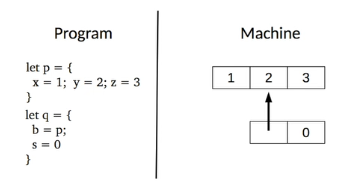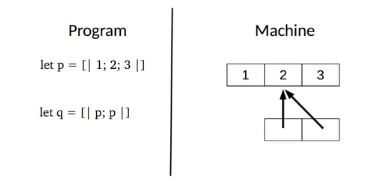# [OCaml MOOC] week2: BASIC DATA STRUCTURES

[TOC]

this week: structure code with types: tuples, records, arrays.

# 1. USER-DEFINED TYPES

### primary use of types: document your code• use `type type_identifier = some_type` to define a new type (`type_identifier` is synonym/abbrevation of `some_type`)
• `type_identifier` must start with lowercase letter
• already known types: `int, bool, string, char` ...
• use `:` to add type annotation to identifiers `let x : some_type = some_expr`
• annotation param/return type of a function: `let f(x: some_type): return_type = some_expr` or `let f x : return_type = some_expr`

example: colors

```# type color = int;;
type color = int
# let red: color = 0;;
val red : color = 0
# let white: color = 1;;
val white : color = 1
# let blue: color = 2;;
val blue : color = 2
```

example: positive integers

```# type positive = int;;
type positive = int
# let abs(x:int) = (if x<0 then -x else x: positive);;
val abs : int -> positive = <fun>
# let abs' (x:int): positive = if x<0 then -x else x;;
val abs' : int -> positive = <fun>
```

### pitfalls

• in the REPL, careful with unintended hiding of type identifiers:
```# type t = int;;
type t = int
# let x: t = 0;;
val x : t = 0
# type t = bool;;
type t = bool
# let f (x: t) = not x;;
val f : t -> bool = <fun>
# let z = f x;;
Error: This expression has type t/1027 = int
but an expression was expected of type t/1029 = bool
```
• limitations of type synonyms⇒ but ocaml has ways to define more precise types to avoid such error statically

# 2. TUPLES: positioned components

Some objects are naturally made of several components, example: 2d point.

```# let origin = (0,0);;
val origin : int * int = (0, 0)
# let positive_range = (max_int, 0);;
val positive_range : int * int = (4611686018427387903, 0)
# let negative_range = (min_int, 0);;
val negative_range : int * int = (-4611686018427387904, 0)
```

name tuples with `type`: use `*` to construct tuple types.

```# type point2D = int * int ;;
type point2D = int * int
# let origin : point2D = (0,0);;
val origin : point2D = (0, 0)
```

### pattern matching: observing components of tuple

• pattern: describe how values are observed by the program.
• appear in let-bindings or in func arguments.
• simplest form of pattern: identifiers

example: `let x = 6*3 in x` can be read as "I observe the value of 63 by naming it as x*"

• ignore some component using wildcard `_`, example: `let _ = 6*3 in 1;;`

example for tuple: `let (x, _) = (5, 2) in x;;` can read as: "I observe the first component of tuple (5,2) by naming it as x, and I ignore the 2nd component"

```# let a = (3,4);;
val a : int * int = (3, 4)
# let (x,_) = a;;
val x : int = 3
# let x_coord (x, _) = x;;
val x_coord : 'a * 'b -> 'a = <fun>
# let y_coord (_, y) = y;;
val y_coord : 'a * 'b -> 'b = <fun>
```

### tuples in the machine

tuple is a block of memory, program holds pointer to the block,pointers can be shared:### structrual equality VS physical equaliity

in ocaml there are 2 types of equalities:

• `=` implies structrual eq — i.e. they have the same content.
• `==` implies physical eq — i.e. they are stored in the same memory location.
```# let x = (1,2)
let y = (1,2)
let z = x;;
val x : int * int = (1, 2)
val y : int * int = (1, 2)
val z : int * int = (1, 2)
# x = y;;
- : bool = true
# x == y;;
- : bool = false
# x == z;;
- : bool = true
```

### pitfalls

• mismatching number of components : can be caught by compiler
• semantic errors:

example: `# let x_coord (x, y) = y;;` can't be caught by compiler → using records.

# 3. RECORDS: naming components

name each components of a tuple → record.

example:

```# type point2D = {x:int ; y:int};;
type point2D = { x : int; y : int; }
# let origin = {x = 0; y = 0};;
val origin : point2D = {x = 0; y = 0}
# let from_tuple (x,y) = {x ; y};;
val from_tuple : int * int -> point2D = <fun>
# let a = from_tuple (4,2) ;;
val a : point2D = {x = 4; y = 2}
# let b = from_tuple (10, 5);;
val b : point2D = {x = 10; y = 5}
# type box = {left_upper: point2D; right_lower: point2D;};;
type box = { left_upper : point2D; right_lower : point2D; }
# let abox = {left_upper=a; right_lower=b};;
val abox : box = {left_upper = {x = 4; y = 2}; right_lower = {x = 10; y = 5}}
# let get_min_xcoord {left_upper = {x}} = x;; (*pattern matching here for the func*)
val get_min_xcoord : box -> int = <fun>
```

decalre a record type:

`type som_type_identifier = {field_name: some_type; ...; field_name: some_type}`

construct a record:
`{field_name = some_expr; ...; field_name = som_expr}`

observe a field:
`some_expr.field_name`

observe several fields: use record patterns:
`{field_name = some_pattern; ...; field_name = some_pattern}`
(don't have to write all fields here)

in the machine: like a tuple, a block of memory### pitfalls: shadowing a field name

if 2 records share some identical field names:

```# type a = {x:int; b:int};;
type a = { x : int; b : int; }
# type b = {y:int; c:int;};;
type b = { y : int; c : int; }
# {x=0; b=2};;
- : a = {x = 0; b = 2}
# type t = {x:bool};;
type t = { x : bool; }
# {x=true};;
- : t = {x = true}
# type u = {x:int};;
type u = { x : int; }
# {x=true};;
Error: This expression has type bool but an expression was expected of type int
```

advice: NOT share field names between records!

# 4. ARRAYS

tuple/record: sizes are statically bounded.

array: dynamically change sequence size,
but all array elements must have same type.

```# let p = [|1;2;3;|];;
val p : int array = [|1; 2; 3|]
# let square x = x * x;;
val square : int -> int = <fun>
# let squares n = Array.init n square;;
val squares : int -> int array = <fun>
# let s1 = squares 5;;
val s1 : int array = [|0; 1; 4; 9; 16|]
```

Std module `Array`: provides functions over arrays

• `some_array = [|some_expr; ...; some_expr|]`
• `Array.make sz val`: takes an int (size of array) and a value to initialize each component of the array (like Array.fill).
• `Array.init sz f`: initial size of array `sz` and a function `f` to initilize each component of array, f takes the index of the component and returns a value.
• `Array.length arr`: returns array size.
• array indexing: `arr.(some_expr:int)`, index: 0 to sz-1
• array patterns: observe several components of array `[|some_expr; ...; some_expr|]` (not very useful)
```# let swap a = [|a.(1); a.(0)|];;
val swap : 'a array -> 'a array = <fun>
# let b = swap [|0;1|];;
val b : int array = [|1; 0|]
# let swap [|x; y|] = [|y; x|];;
Characters 9-28:
Warning 8: this pattern-matching is not exhaustive.
Here is an example of a value that is not matched: [|  |]
val swap : 'a array -> 'a array = <fun>
# let t = swap [|2;1|];;
val t : int array = [|1; 2|]
# let t = swap [|2;1;0|];;
Exception: Match_failure ("//toplevel//", 1, 9).
```

in the machine: a memory block, like a record# 5. CASE STUDY: A SMALL TYPED DATABASE

a toy db for contacts,
3 queries: `insert, delete, search`.

These functions have type: `database -> query_contact -> status * database * contact`

define the phone number/contact/database types:

```type phone_number = int * int * int * int;;

type contact = {
name         : string;
phone_number : phone_number
};;

let nobody = { name = ""; phone_number = (0, 0, 0, 0) };;

type database = {
number_of_contacts : int;
contacts : contact array;
};;
```

implement `make` (to create a database with parameter=max capacity):

```let make max_number_of_contacts =
{
number_of_contacts = 0;
contacts = Array.make max_number_of_contacts nobody
};;
(* Queries are represented by a code and a contact.
- If the code is 0 then the contact must be inserted.
- If the code is 1 then the contact must be deleted.
- If the code is 2 then we are looking for a contact
with the same name in the database. *)
type query = {
code    : int;
contact : contact;
}
```

implement `search`:

```let search db contact =
let rec aux idx =
if idx >= db.number_of_contacts then
(false, db, nobody)
else if db.contacts.(idx).name = contact.name then
(true, db, db.contacts.(idx))
else
aux (idx + 1)
in
aux 0;;
```

implement `insert`:

```let insert db contact =
if db.number_of_contacts >= Array.length db.contacts then
(false, db, nobody)
else
let (status, db, _) = search db contact in
if status then (false, db, contact) else
let cells i =
if i = db.number_of_contacts then contact else db.contacts.(i)
in
let db' = {
number_of_contacts = db.number_of_contacts + 1;
contacts = Array.init (Array.length db.contacts) cells
}
in
(true, db', contact);;
```

implement `delete`:

```let delete db contact =
let (status, db, contact) = search db contact in
if not status then (false, db, contact)
else
let cells i =
let last = db.contacts.(db.number_of_contacts-1) in
if db.contacts.(i).name = contact.name then last
else if i==(db.number_of_contacts-1) then nobody
else db.contacts.(i) in
let db' = {
number_of_contacts = db.number_of_contacts - 1;
contacts = Array.init (Array.length db.contacts) cells
}
in (true, db', contact);;
```

implement an engine function to process all kinds of queries:

```(* Engine parses and interprets the query. *)
let engine db { code ; contact } =
if code = 0 then insert db contact
else if code = 1 then delete db contact
else if code = 2 then search db contact
else (false, db, nobody);;
```

Remarks:

This is purely functional, a new db is created each time a query is processed.

#### Part 3 of series «Introduction to Functional Programming in OCaml»：

comments powered by Disqus